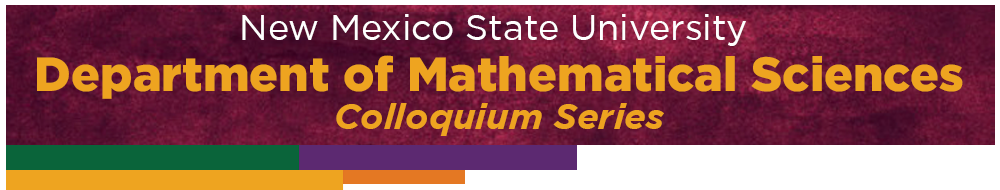####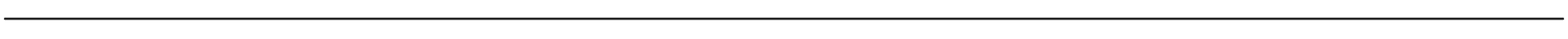-- For direct zoom link click here

Spring 2023

 02/24: Alexander Shen Title: Information Inequalities Abstract: The intuitive notion of "amount of information" can be formalized in may ways. The algorithmic information (Kolmogorov complexity) theory says that the amount of information in a finite object x is the minimal length of a program that produces x. Some intuitive properties of information (say, the amount of information in pair (x,y) is bounded by the sum of the amounts of information in x and y) can be proven.  Another way to measure information is suggested by Shannon information theory. It turns out that the "laws of information theory" (understood as linear inequalities) are the same for both cases (Romashchenko), and the same class of inequalities appears in group theory, database theory, geometric dimension theory. A survey of these results will be given. 01/25: Yairon Cid-Ruiz Title: Multidegrees at the crossroads of Algebra, Geometry, and Combinatorics. Abstract: The concept of multidegrees provides the right generalization of the degree of a projective variety to a multiprojective setting. The study of multidegrees goes back to seminal work by van der Waerden in 1929. We will slowly introduce the notion of multidegrees of a multiprojective variety. A complete characterization of the positivity of multidegrees will be presented. Finally, we will apply our methods on multidegrees to settle a conjecture of Monical, Tokcan, and Yong for double Schubert polynomials and a particular case of a conjecture of the same authors for Grothendieck polynomials. We will report on joint works with Federico Castillo, Binglin Li, Fatemeh Mohammadi, Jonathan Montaño, and Naizhen Zhang. 01/23: Boyu Li Title: Analysis on Semigroups Through the Lens of Dilation Theory Abstract: The analysis of algebraic structures using bounded linear operators on Hilbert spaces traces back to the pioneering work of Murray and von Neumann. For example, the abstract harmonic analysis of groups relates various properties of a group to its unitary representations. For semigroups, however, the lack of inverse leads to much richer classes of representations. These representations are often interrelated via dilation theorems. In this talk, I will briefly go over the history of the analysis of semigroups, with a focus on the role of dilation theory. I will then explain how our recent dilation theorem on semigroup dynamical systems unifies many earlier results in dilation theory. This is partly based on joint work with Marcelo Laca. 01/20: Michael DiPasquale Title: A Bridge Between the Algebra and Geometry of Hyperplane Arrangements Abstract: A hyperplane arrangement is a union of codimension one linear spaces.  These simple objects provide fertile ground for interactions between combinatorics, algebra, algebraic geometry, topology, and group actions.  The combinatorics of an arrangement is encoded by the pattern of intersections among the hyperplanes, called its intersection lattice.  On the other hand, a key algebraic object is the module of vector fields tangent to the arrangement, called the module of logarithmic derivations, which was introduced by Saito in 1980 to study the singularities of hypersurfaces.  An enduring mystery in the theory of hyperplane arrangements is which algebraic properties of the module of logarithmic derivations can be determined from the intersection lattice, and which properties depend fundamentally on the geometry (i.e. the exact hyperplanes).  At the center of this mystery is Terao's conjecture, which proposes that the algebraic property of freeness can be determined only from the intersection lattice.  In this talk we explain how rigidity of planar frameworks (dating back to Maxwell) plays a key role in connecting the geometry and algebra of line arrangements in the projective plane. This is joint work with Jessica Sidman and Will Traves. 01/18: Timothy Rainone Title: Paradoxical decompositions, dichotomy, and Finite-Approximability in Operator Algebras Abstract: Notions of finiteness are prevalent in mathematics, not least in operator algebras. For instance, with matrix algebras we observe Dedekind-finiteness; that is every isometry is unitary. This also holds for large classes of C*-algebras as well as their stabilization. Such algebras are called stably finite. At the opposite end of the spectrum are the purely infinite C*-algebras whose internal structure admit paradoxical decompositions; especially when the algebras in question are crossed-product constructions. In this talk we will study the stably finite/purely infinite dichotomy for certain C*-algebras that arise from dynamical systems. We will then move our discussion to matricial field (MF) C*-algebras; a subclass of stably finite algebras that admit external matrix approximations. The C*-analogue of the Connes Embedding Conjecture proposes that every stably finite C*-algebra should admit such an approximation. We show that is is indeed the case for large classes of crossed products of nuclear algebras by free groups. This talk is aimed at a general math audience; we will carefully introduce the theory of C*-algebras as well as all the notions alluded to above. 01/16: Alessandra Costantini Title: Rees Algebras: An Algebraic Tool to Study Singularities Abstract: How many tangent lines does a plane curve have at a given point? And how can we effectively solve polynomial equations with integral coefficients? These seemingly unrelated problems from algebraic geometry and number theory can be both solved using tools from abstract algebra, through the notion of Rees algebras.  In this talk, I will first discuss the fundamental role of Rees algebras in the study of commutative rings and singularities of algebraic varieties. I will then focus on the problem of describing Rees algebras in terms of generators and relations, presenting some of my recent results on this topic, which were obtained using techniques from algebraic combinatorics. 01/13: Sudan Xing Title: Dual Orlicz-Minkowski Problem Abstract: The classical Minkowski problem is a central problem in convex geometry which asks that given a nonzero finite Borel measure, what are the necessary and sufficient conditions on this measure such that it equals to the surface area measure of a convex body. In this talk, I will present the general dual extension of the classical Minkowski problem—the generalized dual Orlicz-Minkowski problem.

Previous Colloquium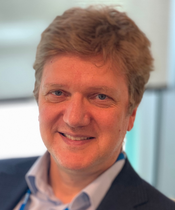11/11/2022: Vakhtang Putkaradze Title: Variational Approach to Porous Media: Equations of Motion, Muscle Action and Thermodynamics Abstract: Porous media presents a highly complex example of fluid-structure interactions where a deforming elastic matrix interacts with the fluid. Many biological organisms are comprised of deformable porous media, with additional complexity of a muscle acting on the matrix. Using geometric variational methods, we derive the equations of motion of a for the dynamics of both the passive and active porous media. The use of variational methods allows to incorporate both the muscle action and incompressibility of the fluid and the elastic matrix in a consistent, rigorous framework. We also derive conservation laws for the motion, perform numerical simulations and show the possibility of self-propulsion of a biological organism due to particular running wave-like application of the muscle stress. We also discuss variational derivation for equations of porous media from the point of view of variational thermodynamics, leading to conclusions about thermodynamically consistent functional forms of friction forces and stresses acting on the media. Joint work with F. Gay-Balmaz and T. Farkhutdinov.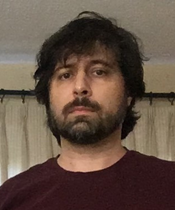11/4/2022: Mihai Popa Title: Entry Permutations, Limit Distributions and Asymptotic Free Independence for Some Relevant Classes of Random Matrices Abstract:  In the study of large random matrices, the notion of free independence is a suitable analogue to the notion of independence from the study of commutative random variables. In particular, since 1980s, large classes of random matrices have been shown to be asymptotically free from random matrices with entries independent from their entries. Some years ago, together with J.A. Mingo, we showed the (at that time, surprising) result that, under reasonable technical assumptions, ensembles of unitarily invariant random matrices are asymptotically free from their transposes. This fact brought the natural question "How special is the transpose?". That is, given an ensemble of random matrices $( A_N)_N$ and a sequence of entry permutations $( \sigma_N)_N$ can we formulate conditions such that $(A_N)_N$, the initial ensemble, and $(A_N^{\sigma_N)_N$, the ensemble consisting of matrices with permuted entries, are asymptotically free? Also, what are the possible limit distributions for the matrices with permuted entries? The lecture will present some recent progresses as well as some still open questions in this topic.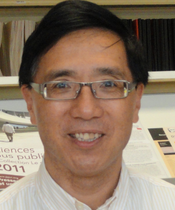10/28/2022: Quanhua Xu Title: Maximal Inequalities in Noncommutative Analysis Abstract: Maximal inequalities are of paramount importance in analysis. Here “analysis” is understood in a wide sense and includes functional/harmonic analysis, ergodic theory and probability theory. Consider, for instance, the following two fundamental examples: Click here We will consider in this survey talk the analogues of these classical inequalities in noncommutative analysis. Then the usual Lp-spaces are replaced by noncommutative Lp-spaces. The theory of noncommutative martingale/ergodic inequalities was remarkable developed in the past 20 years. Many classical results were successfully transferred to the noncommutative setting. This theory has fruitful interactions with operator spaces, quantum probability and noncommutative harmonic analysis. We will discuss some of these noncommutative results and explain certain substantial difficulties in proving them.10/21/2022: Andre Kornell Title: Four Categories of Hilbert Spaces Abstract: Hilbert spaces over the real or the complex numbers form monoidal categories in two distinct ways. We can take a morphism of Hilbert spaces to be a bounded operator or a contractive operator. All four categories can be defined axiomatically without any reference to the real or the complex numbers. The proof appeals to Soler's theorem, the Hellinger-Toeplitz theorem, and the Russo-Dye theorem, as well as to a body of research on dagger categories. Joint work Chris Heunen and Nesta van der Schaaf.10/07/2022: Joel Lucero-Bryan Title: Modal Logic of Topology: An Introduction Abstract: In topological semantics of modal logic, the modal box is interpreted as topological interior (and hence diamond as closure). This is one of the oldest semantics for modal logic, dating back to the late 1930s and early 1940s. One of the key early results is the 1944 theorem of McKinsey and Tarski that the modal logic of any crowded (separable) metric space is Lewis's well known modal system S4. In the last 30 years, utilizing the machinery of relational semantics, new techniques have been developed that paved the way to obtaining further results that both generalize the McKinsey-Tarski theorem in several directions as well as provide interesting connections with other areas of mathematics. Our aim is to review some of these results. The talk will include a hands-on portion addressing one of the key features of topological semantics: obtaining finite configurations as continuous and open images of such well-known topological spaces as the real line. Time permitting, I will also present some new topological invariants that arise via these considerations.09/30/2022: John C. Dallon Title: Techniques of Analyzing Stochastic Differential Equation Systems Abstract: In this talk I will present a force based mathematical model of mesenchymal cell motion where adhesion sites are randomly placed and have a probabilistically determined lifespan. Numerical simulations and theoretical results will be discussed and compared to experimental data. The goal is to understand how focal adhesion dynamics affect cell motion.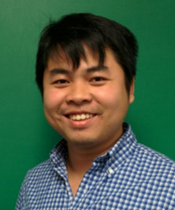09/16/2022: Tuan Phan Title: Modeling Mesenchymal Cell Motion - Force, Speed and the Role of Focal Adhesion Lifetimes Abstract: In this talk, I will discuss two techniques to analyze theoretically a stochastic differential equation (SDE) system that often arises in modeling biological and medical problems. The first one is to construct stochastic Lyapunov functions for proving the existence of a unique stationary distribution of a SDE system in the interior of its invariant domain. The second one is to analyze the dynamics of a SDE system on the boundary of its invariant domain. The latter technique has some advantages and can provide sharp conditions for the extinction and persistence of a SDE system, which is an important concern in mathematical biology. I will use a cholera epidemic model and human papilloma virus model to demonstrate how to use and combine these two techniques. I will keep technique low and emphasize ideas.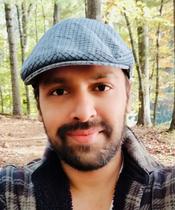09/02/2022: Prasit Bhattacharya Title: Rabbit holes of Spheres Abstract: Many intricate and bizarre patterns have been discovered while studying the homotopy groups of spheres. These groups have been the subject of study for more than a century yet they are mostly unknown. This talk will discuss many interesting patterns, especially the ones that led to chromatic homotopy theory, a modern subject which sheds light on large scale patterns within the stable homotopy groups of spheres.